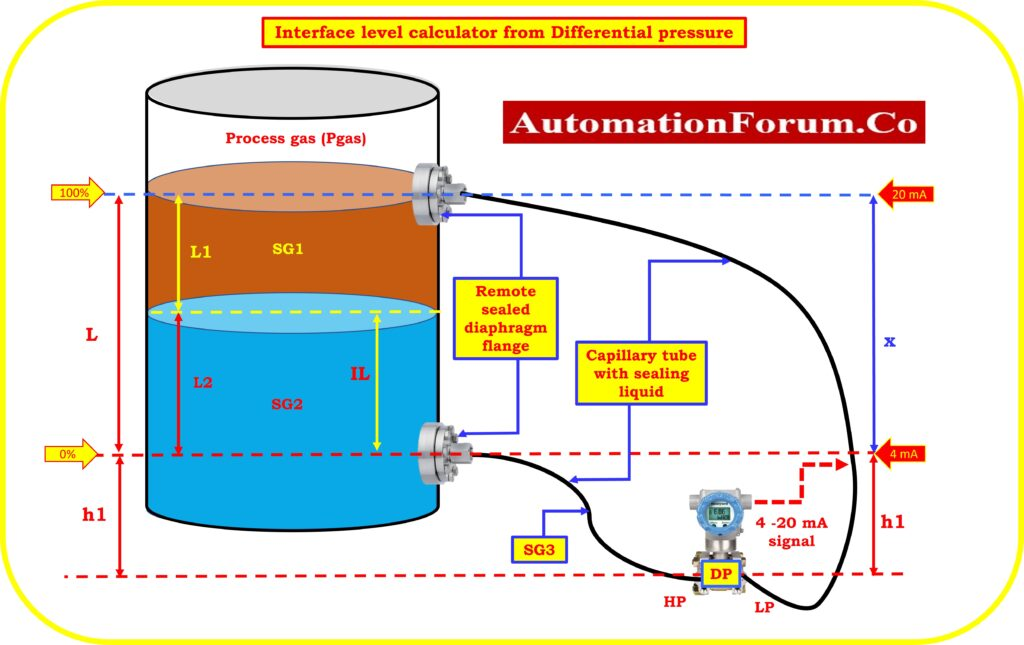# Interface level calculator from Differential pressure (DP)## How do you calculate interface level?

The reading of the Differential pressure (DP) expressed as a percentage of the span can be used to pinpoint the location of the interface between two fluids that have been mixed together.

The formula that is used to compute the interface is as follows:

IL= {(DP- LRV)/S} *L

IL = Interface level

LRV = Lower range value of the DP level transmitter

DP = Measured differential pressure by the transmitter

S = Span value calibrated transmitter (URV – LRV)

L = Engineering range of the liquid interface level

LI = Lighter liquid level need to be measured

L2 = Heavier liquid level need to be measured

## Interface level calculator

The below calculator used to calculate the interface level from the differential pressure indicated by the transmitter